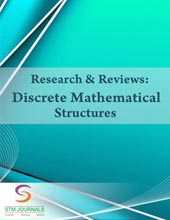﻿ Journal of Discrete Mathematics (STM Journals)# Research & Reviews: Discrete Mathematical Structures

• eISSN: 2394-1979#### Avail Online Certificate for article along with DOI number## Editor, Reviewer and AuthorResearch & Reviews: Discrete Mathematical Structures (RRDMS): Discrete mathematical structures journal deals with discrete objects. Discrete objects are those which are separated from each other like integers, rational numbers, automobiles, houses, peoples etc. are all discrete objects. Some of the major reasons that we adopt discrete mathematics are. We can handle infinity or large quantity and indefiniteness with them which results from formal approaches are reusable.

Focus and Scope Covers• Mathematical induction
• Logic and boolean algebra
• Set theory
• Relations and functions
• Sequences and series
• Algorithms and theory of computation
• Number theory
• Matrix theory
• Induction and recursion
• Counting and discrete probability
• Graph theory (trees)
• Calculus of finite differences, discrete calculus or discrete analysis
• Game theory, decision theory, utility theory, social choice theory
• Discrete analogues of continuous mathematics
• Hybrid discrete and continuous mathematics

All contributions to the journal are rigorously refereed and are selected on the basis of quality and originality of the work. The journal publishes the most significant new research papers or any other original contribution in the form of reviews and reports on new concepts in all areas pertaining to its scope and research being done in the world, thus ensuring its scientific priority and significance.

## Subscribe to this Journal